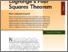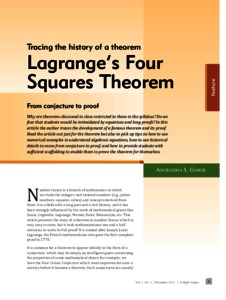# Tracing the history of a theorem Lagrange’s four squares theorem

Garge, Anuradha S. (2012) Tracing the history of a theorem Lagrange’s four squares theorem. At Right Angles, 1 (2). pp. 5-9.Preview
Text - Published Version

## Abstract

Why are theorems discussed in class restricted to those in the syllabus? Do we fear that students would be intimidated by equations and long proofs? In this article the author traces the development of a famous theorem and its proof. Read the article not just for the theorem but also to pick up tips on how to use numerical examples to understand algebraic equations, how to use historical details to move from conjecture to proof, and how to provide students with sufficient scaffolding to enable them to prove the theorem for themselves.

Item Type: Articles in APF MagazinesView Item